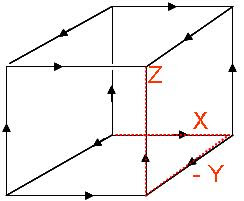## M Theory Lesson 110

The connection between mass matrices and Fourier transforms leads to endless musing over the important work of Kapranov! Recall that a path on a cubical lattice is represented by a monomial in three letters, such as $X(-Y)Z$.To begin with we may consider positive paths in the positive octant (ie. 3d Young diagrams, which don’t backtrack) and forget the minus signs. If the path taken to the target point was irrelevant, a commutative monomial $XYZ$ would denote the target point without its path history. Only non-commutative monomials describe all possible paths from the source to the target. On considering $3 \times 3 \times 3$ cubes, homogeneous monomials of total degree 9 describe the paths. The homogeneity follows from the symmetry of subdivision.

A path integral for a fixed source and target may have contributions from paths of varying length, depending on the number of subdivisions. The shortest possible path here is of length 3. Recall that in one dimension, the number of subdivisions $n$ is associated to the size of a circulant matrix, associated to the position space $\mathbb{Z}_n$. But this almost trivial one dimensional case somehow encoded the three dimensions of space! A truly three dimensional Fourier transform, with a six dimensional phase space, would contain much more physical information.

Aside: Carl Brannen’s new site has a sidebar button.

## 1 Response so far »

1. 1### Doug said,

Hi Kea,

Speculation:

One might be able to circumscribe a 3-ball around your pictured cube.

Then one may be able to circumscribe a larger cube around this 3-ball, leading to a situation similar to that of Archimedes with polygons and a circle.

Then these two cubes may be connected like a tesseract which has some popularity in science fiction?

see image gallery section of this wiki entry
http://en.wikipedia.org/wiki/Tesseract
or
http://mathworld.wolfram.com/Tesseract.html

The tesseract “is one of the six regular polychora.”
http://mathworld.wolfram.com/
RegularPolychoron.html